# Introduction to splines2

#### 2020-07-13

The splines2 package provides functions constructing a variety of regression spline bases that are not available from the splines package shipped with base R. Most functions have a very similar user interface with the function splines::bs(). To be more specific, it provides function constructing B-splines, integral of B-splines, M-splines and its integral (I-splines), convex splines (C-splines), generalized Bernstein polynomials, and their derivatives of given order. Compared with the splines package, splines2 allows piece-wise constant basis for B-splines and provides a more user-friendly interface for their derivatives with consistent handling on NA’s. Most of the implementations has been (re)written in C++ with the help of Rcpp and RcppArmadillo since v0.3.0, which helps boost the computational performance.

In the remaining of this vignette, we introduce the basic usage of most functions in the package through examples. The details of function syntax are available in the package manual and thus will be not discussed.

## B-splines with their integrals and derivatives

Function bSpline() provides B-spline basis matrix and allows degree = 0 for piece-wise constant bases, which extends the bs() function in package splines with a better computational performance. One example of linear B-splines with two internal knots is given as follows:

library(splines2)
knots <- c(0.3, 0.5, 0.6)
x <- seq(0, 1, 0.01)
bsMat <- bSpline(x, knots = knots, degree = 1, intercept = TRUE)
matplot(x, bsMat, type = "l", ylab = "y")
abline(v = knots, lty = 2, col = "gray")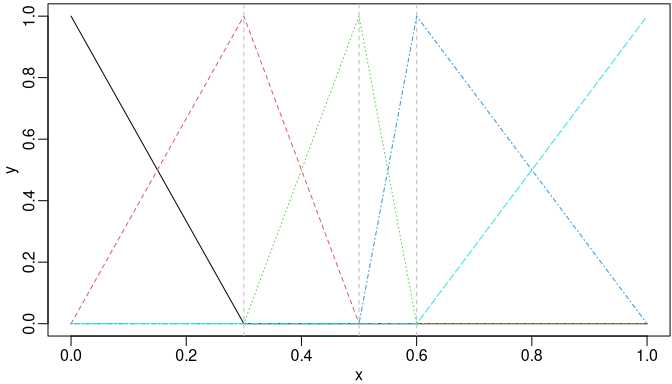B-splines of degree one with two internal knots.

The close-form recursive formula of B-spline integrals and derivatives given by De Boor (1978) is implemented in function ibs() and dbs(), respectively. Two toy examples are given as follows:

ibsMat <- ibs(x, knots = knots, degree = 1, intercept = TRUE)
par(mfrow = c(1, 2))
matplot(x, bsMat, type = "l", ylab = "y")
abline(v = knots, h = 1, lty = 2, col = "gray")
matplot(x, ibsMat, type = "l", ylab = "y")
abline(v = knots, h = c(0.15, 0.2, 0.25), lty = 2, col = "gray")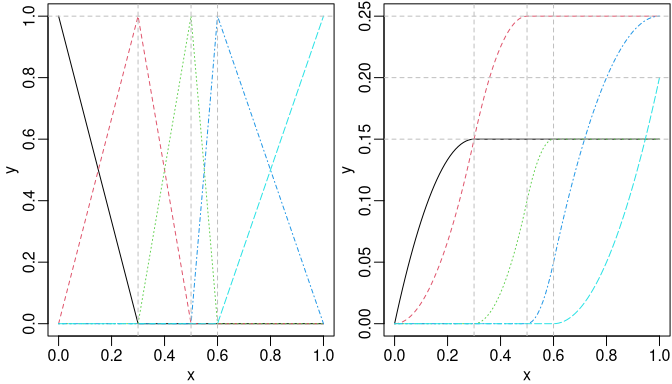Piecewise linear B-splines (left) and their integrals (right).

bsMat <- bSpline(x, knots = knots, intercept = TRUE)
dbsMat <- dbs(x, knots = knots, intercept = TRUE)
par(mfrow = c(1, 2))
matplot(x, bsMat, type = "l", ylab = "y")
abline(v = knots, lty = 2, col = "gray")
matplot(x, dbsMat, type = "l", ylab = "y")
abline(v = knots, lty = 2, col = "gray")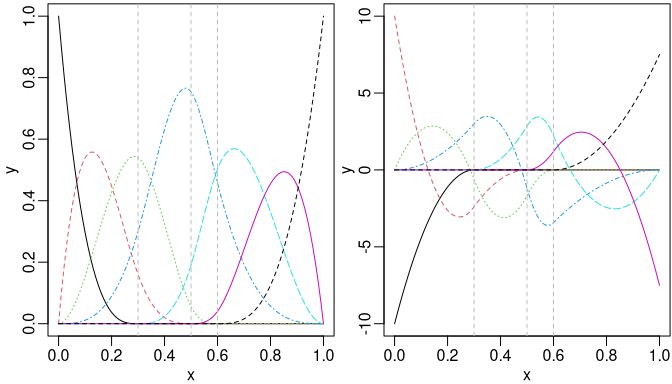Cubic B-splines (left) and their first derivative (right).

We may also obtain the derivatives easily by the deriv() method as follows:

is_equivalent <- function(a, b) {
all.equal(a, b, check.attributes = FALSE)
}
stopifnot(is_equivalent(dbsMat, deriv(bsMat)))

## M-splines using mSpline()

M-splines (Ramsay 1988) can be considered as a normalized version of B-splines with unit integral within boundary knots. An example given by Ramsay (1988) was a quadratic M-splines with three internal knots placed at 0.3, 0.5, and 0.6. The boundary knots by default are the range of the data x, thus 0 and 1 in this example.

msMat <- mSpline(x, knots = knots, degree = 2, intercept = TRUE)
matplot(x, msMat, type = "l", ylab = "y")
abline(v = knots, lty = 2, col = "gray")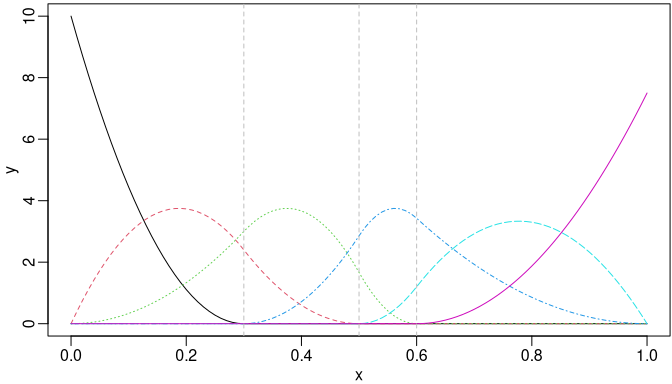Quadratic M-splines with three internal knots.

The derivative of given order of M-splines can be obtained by specifying a positive integer to argument dervis of mSpline(). Also, for an existing mSpline object generated by mSpline(), the deriv() method can be used conveniently. For example, the first derivative of the M-splines given in last example can be obtained equivalently as follows:

dmsMat1 <- mSpline(x, knots = knots, degree = 2, intercept = TRUE, derivs = 1)
dmsMat2 <- deriv(msMat)
stopifnot(is_equivalent(dmsMat1, dmsMat2))

## I-splines using iSpline()

I-splines (Ramsay 1988) are simply the integral of M-splines and thus monotonically non-decreasing with unit maximum value. A monotonically non-decreasing (non-increasing) function can be fitted by a linear combination of I-spline bases with non-negative (non-positive) coefficients plus a constant, where the coefficient of the constant is unconstrained.

The example given by Ramsay (1988) was the I-splines corresponding to that quadratic M-splines with three internal knots placed at 0.3, 0.5, and 0.6. Notice that the degree of I-splines is defined from the associated M-splines instead of their own polynomial degree.

isMat <- iSpline(x, knots = knots, degree = 2, intercept = TRUE)
matplot(x, isMat, type = "l", ylab = "y")
abline(h = 1, v = knots, lty = 2, col = "gray")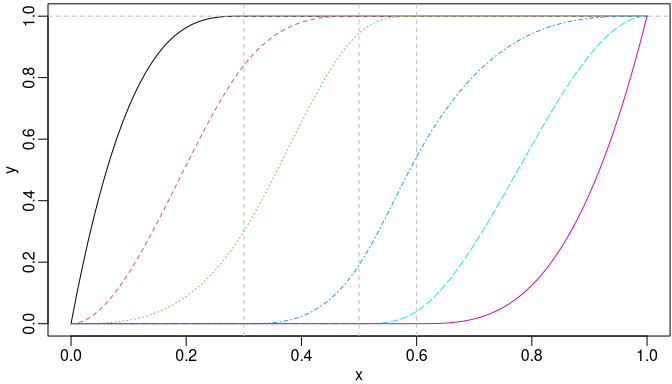I-splines of degree two with three internal knots.

The corresponding M-spline basis matrix can be obtained easily as the first derivatives of the I-splines by the deriv() method.

stopifnot(is_equivalent(msMat, deriv(isMat)))

We may specify the derivs = 2 in the deriv() method for the second derivatives of the I-splines, which are equivalent to the first derivatives of the corresponding M-splines.

dmsMat3 <- deriv(isMat, 2)
stopifnot(is_equivalent(dmsMat1, dmsMat3))

## C-splines using cSpline

Convex splines (Meyer 2008) called C-splines are scaled integrals of I-splines with unit maximum value at the right boundary knot. Meyer (2008) applied C-splines to shape-restricted regression analysis. The monotone (non-decreasing) property of I-spines ensures the convexity of C-splines. A convex regression function can be estimated using linear combinations of the C-spline bases with non-negative coefficients, plus an unconstrained linear combination of a constant and an identity function $$g(x)=x$$. If the underlying regression function is both increasing and convex, the coefficient on the identity function is restricted to be non-negative as well.

We may specify the argument scale = FALSE in function cSpline() to disable the scaling of the integrals of I-splines. Then the actual integrals of the corresponding I-splines will be returned. If scale = TRUE (by default), each C-spline basis is scaled to have unit height at the right boundary knot.

csMat1 <- cSpline(x, knots = knots, degree = 2, intercept = TRUE)
matplot(x, csMat1, type = "l", ylab = "y")
abline(h = 1, v = knots, lty = 2, col = "gray")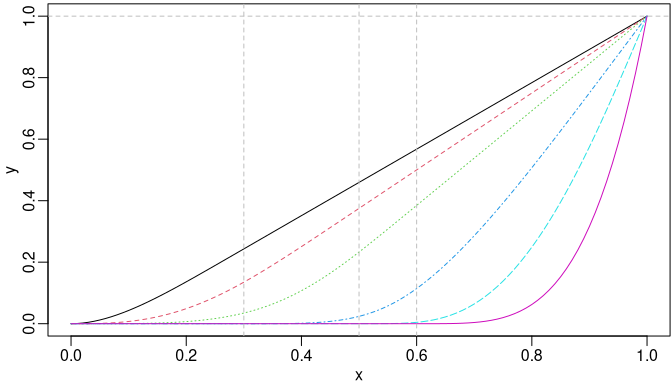C-splines of degree two with three internal knots.

Similarly, the deriv() method can be used to obtain the derivatives. A nested call of deriv() is supported for derivatives of order greater than one. However, the argument derivs of the deriv() method can be specified directly for a better computational performance. For example, the first and second derivatives can be obtained by the following equivalent approaches, respectively.

csMat2 <- cSpline(x, knots = knots, degree = 2, intercept = TRUE, scale = FALSE)
stopifnot(is_equivalent(isMat, deriv(csMat2)))
stopifnot(is_equivalent(msMat, deriv(csMat2, 2)))
stopifnot(is_equivalent(msMat, deriv(deriv(csMat2))))

## Generalized Bernstein Polynomials

The Bernstein polynomials has also been applied to shape-constrained regression analysis (Wang and Ghosh 2012). The $$i$$-th basis of the generalized Bernstein polynomials of degree $$n$$ over $$[a, b]$$ is defined as follows: $B_i^n(x)=\frac{1}{(b-a)^n}{n\choose i}(x-a)^i (b-x)^{n-i},~0\le i\le n,$ where $$a\le x\le b$$. Obviously, it reduces to Bernstein polynomials defined over $$[0, 1]$$ when $$a = 0$$ and $$b = 1$$.

We may obtain the basis matrix of the generalized using the function bernsteinPoly(). For example, the Bernstein polynomials of degree 4 over [0, 1] and is generated as follows:

x1 <- seq.int(0, 1, 0.01)
x2 <- seq.int(- 1, 1, 0.01)
bpMat1 <- bernsteinPoly(x1, degree = 4, intercept = TRUE)
bpMat2 <- bernsteinPoly(x2, degree = 4, intercept = TRUE)
par(mfrow = c(1, 2))
matplot(x1, bpMat1, type = "l", ylab = "y")
matplot(x2, bpMat2, type = "l", ylab = "y")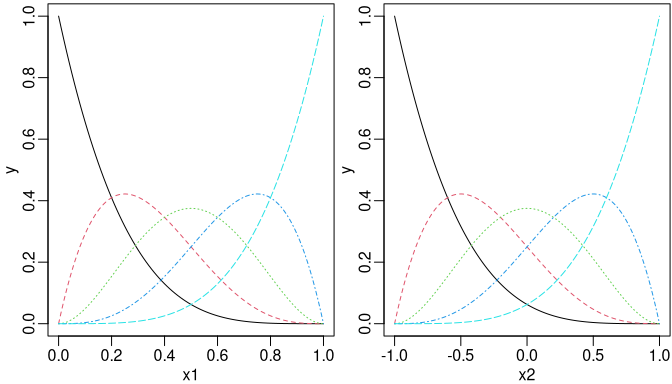Bernstein polynomials of degree 4 over [0, 1] (left) and the generalized version over [- 1, 1] (right).

In addition, we may specify integral = TRUE or derivs = 1 in bernsteinPoly() for their integrals or first derivatives, respectively.

ibpMat1 <- bernsteinPoly(x1, degree = 4, intercept = TRUE, integral = TRUE)
ibpMat2 <- bernsteinPoly(x2, degree = 4, intercept = TRUE, integral = TRUE)
dbpMat1 <- bernsteinPoly(x1, degree = 4, intercept = TRUE, derivs = 1)
dbpMat2 <- bernsteinPoly(x2, degree = 4, intercept = TRUE, derivs = 1)
par(mfrow = c(2, 2))
matplot(x1, ibpMat1, type = "l", ylab = "y")
matplot(x2, ibpMat2, type = "l", ylab = "y")
matplot(x1, dbpMat1, type = "l", ylab = "y")
matplot(x2, dbpMat2, type = "l", ylab = "y")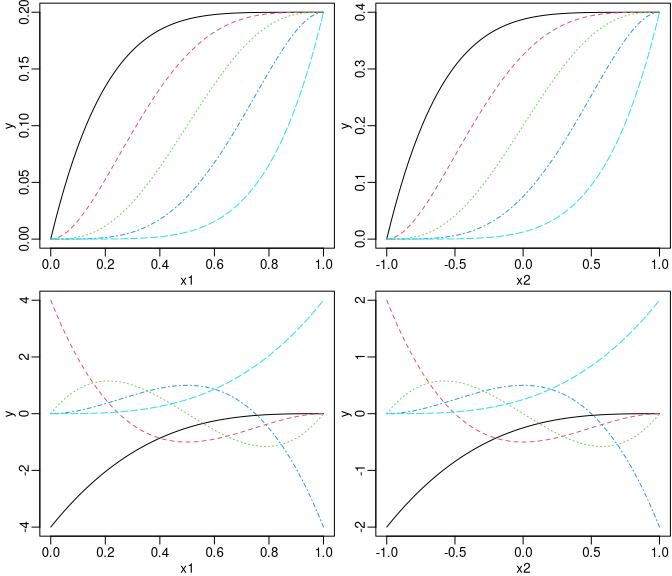Similarly, we may also use the deriv() method to get derivatives of an existing bernsteinPoly object.

stopifnot(is_equivalent(dbpMat1, deriv(bpMat1)))
stopifnot(is_equivalent(dbpMat2, deriv(bpMat2)))
stopifnot(is_equivalent(dbpMat1, deriv(ibpMat1, 2)))
stopifnot(is_equivalent(dbpMat2, deriv(ibpMat2, 2)))

## Evaluation on New Values by predict

The methods for splines2 objects dispatched by generic function predict will be useful if we want to evaluate the spline object at possibly new $$x$$ values. For instance, we may evaluate the value of I-splines object in previous example at 0.275, 0.525, and 0.8, respectively, as follows:

new_x <- c(0.275, 0.525, 0.8)
names(new_x) <- paste0("x=", new_x)
predict(isMat, new_x)
##                 1         2         3         4        5     6
## x=0.275 0.9994213 0.7730556 0.2310764 0.0000000 0.000000 0.000
## x=0.525 1.0000000 1.0000000 0.9765625 0.2696429 0.000625 0.000
## x=0.8   1.0000000 1.0000000 1.0000000 0.9428571 0.580000 0.125

Technically speaking, the methods take all information needed, such as knots, degree, intercept, etc., from attributes of the original objects and call the corresponding function automatically for those new $$x$$ values. Therefore, the predict methods will not be applicable if those attributes are somehow lost after some operations.

## Reference

De Boor, Carl. 1978. A Practical Guide to Splines. Vol. 27. New York: springer-verlag. https://doi.org/10.1002/zamm.19800600129.

Meyer, Mary C. 2008. “Inference Using Shape-Restricted Regression Splines.” The Annals of Applied Statistics, 1013–33. https://doi.org/10.1214/08-AOAS167.

Ramsay, J O. 1988. “Monotone Regression Splines in Action.” Statistical Science, 425–41. https://doi.org/10.1214/ss/1177012761.

Wang, Jiangdian, and Sujit K. Ghosh. 2012. “Shape Restricted Nonparametric Regression with Bernstein Polynomials.” Computational Statistics & Data Analysis 56 (9): 2729–41.